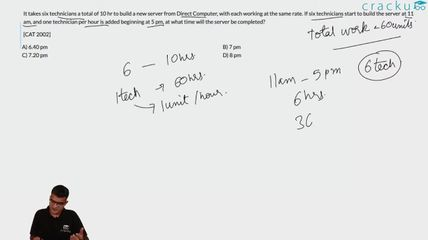Question 48

# It takes six technicians a total of 10 hr to build a new server from Direct Computer, with each working at the same rate. If six technicians start to build the server at 11 am, and one technician per hour is added beginning at 5 pm, at what time will the server be completed?[CAT 2002]

Solution

Let the work done by each technician in one hour be 1 unit.
Therefore, total work to be done = 60 units.
From 11 AM to 5 PM, work done = 6*6 = 36 units.
Work remaining = 60 - 36 = 24 units.
Work done in the next 3 hours = 7 units + 8 units + 9 units = 24 units.
Therefore, the work gets done by 8 PM.

### View Video Solution